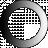# American Dictionary of the English Language

Dictionary Search

### Triangle

TRI'ANGLE, noun [Latin triangulum; tres, tria, three, and angulus, a corner.] In geometry, a figure bounded by three lines, and containing three angles. The three angles of a triangle are equal to two right angles, or the number of degrees in a semicircle.

If the three lines or sides of a triangle are all right, it is a plane or rectilinear triangle

If all the three sides are equal, it is an equilateral triangle

If two of the sides only are equal, it is an isosceles or equicrural triangle

If all the three sides are unequal, it is a scalene or scalenous triangle

If one of the angles is a right angle, the triangle is rectangular.

If one of the angles is obtuse, the triangle is called obtusangular or amblygonous.

If all the angles are acute, the triangle is acutangular or oxygonous.

If the three lines of a triangle are all curves, the triangle is said to be curvilinear.

If some of the sides are right and others curve, the triangle is said to be mixtilinear.

If the sides are all arcs of great circles of the sphere, the triangle is said to be spherical.